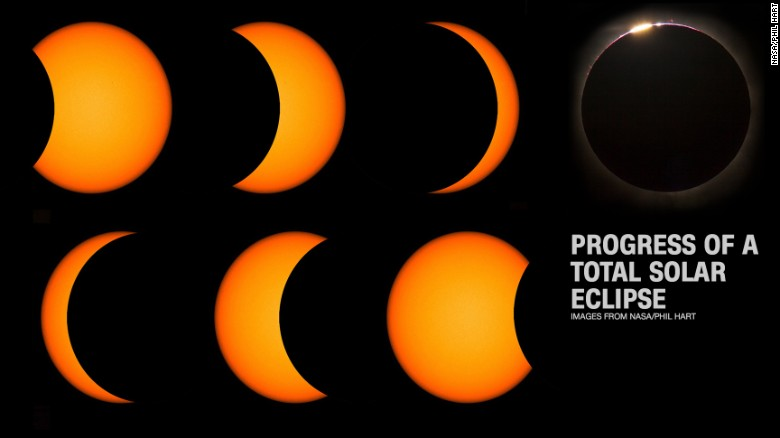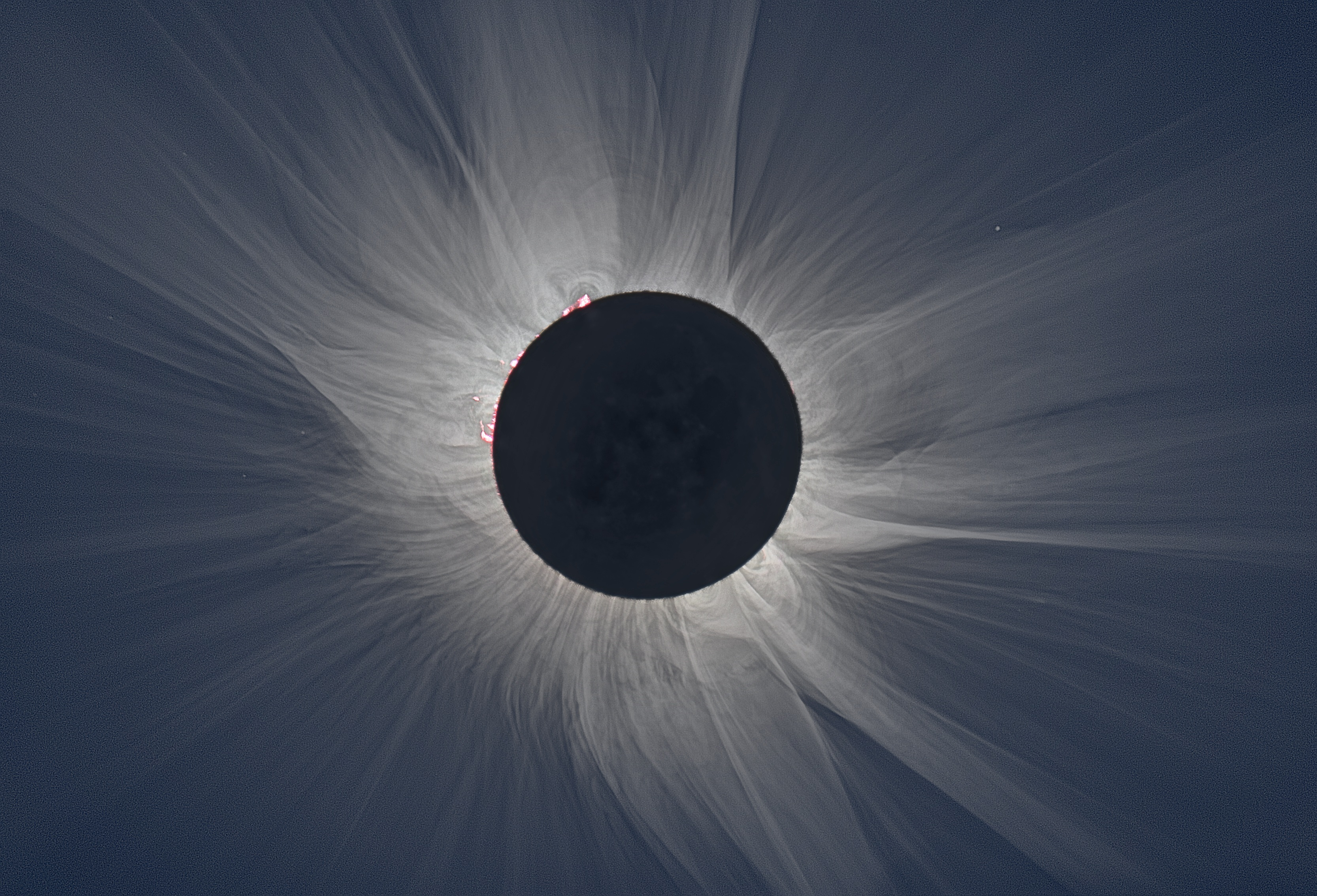# Coincidences and the stealthiness of the Calculus of ProbabilitiesYou know this story (or something similar) from your own life. I was walking from my parked car to the convenience store to purchase a couple of bottles of sparkling water. As I walked there, I noticed a car with the number 1966 – that’s the year I was born! This must be a coincidence – today must be a lucky day!

There are other coincidences, numerical or otherwise. Carl Sagan, in one of his books mentions a person that thought of his mother the very day she passed away in a different city. He (this person) was convinced this was proof of life after/before/during death.

There are others in the Natural World around us (I will be writing about the “Naturalness” idea in the future) – for eclipse aficionados, there is going to be a total solar eclipse over a third of the United States on the 21st of August 2017. It is a coincidence that the moon is exactly the right size to completely cover the sun (precisely – see eclipse photos from NASA below)Isn’t is peculiar that the moon is exactly the right size? For instance, the moon has other properties – for instance, the face of the moon that we see is always the same face. Mercury does the same thing with the Sun – it also exhibits the same face to the Sun. This is well understood as a tidal effect of a small object in the gravitational field of a large neighbor. There’s an excellent Wikipedia article about this effect and I well explain it further in the future. But there is no simple explanation for why the moon is the right size for total eclipses. It is not believed to be anything but an astonishing coincidence. After all, we have 6000 odd other visible objects in the sky that aren’t exactly eclipsed by any other satellite, so why should this particular pair matter, except that they provide us much-needed heat and light?

The famous physicist Paul Dirac discovered an interesting numerical coincidence based on some other numerology that another scientist called Eddington was obsessed with. It turns out that a number of (somewhat carefully constructed) ratios are of the same order of magnitude – basically remember the number$10^{40}$!

• The ratio of the electrical and gravitational forces between the electron and the proton ($\frac{1}{4 \pi \epsilon_0} e^2$ vs.$G m_p m_e$) is approximately$10^{40}$
• The ratio of the size of the universe to the electron’s Compton wavelength, which is the de Broglie wavelength of a photon of the same energy as the electron –$10^{27}m \: vs \: 10^{-12} m \: \approx 10^{39}$

On the basis of this astonishing coincidence, Dirac made the startling observation that this could indicate (since the size of the universe is related to it’s age) the value of$G$ would fall with time (why not$e$ going up with time, or something else?). Whereas precision experiments in measuring the value of$G$ are beginning now, there would have been cosmological consequences if$G$ had indeed behaved as$1/t$ in the past! For this reason, people discount this “theory” these days.

I heard of another coincidence recently – the value of the quantity$\frac {c^2}{g}$, where$c$ is the speed of light and$g$ is the acceleration due to gravity at the surface of the earth ($9.81 \frac{m}{s^2}$), is very close to$1$ light year. This implies (if you put together the formulas for$g$, and$1$ light year), a relationship between the masses of the earth, the sun, the earth’s radius and the earth-moon distance!

The question with coincidences of any sort is that it is imperative to separate signal from noise. And this is why some simple examples of probability are useful to consider. Let’s understand this.

If you have two specific people in mind, the probability of both having the same birthday is$\frac{1}{365}$ – there are 365 possibilities for the second person’s birthday and only 1 way for it to match the first person’s birthday.

If, however, you have$N$ people and you ask for$any$ match of birthdays, there are$\frac {N(N-1)}{2}$ pairs of people to consider and you have a substantially higher probability of a match. In fact, the easy way to calculate this is to ask for the probability of$NO$ matches – that is$\frac {364}{365} \times \frac {363}{365} \times ... \frac {365 - (N-1)}{365}$, which is$\frac {364}{365}$ for the first non-match, then$\frac {363}{365}$ from the second non-match for the third person and so on. Then subtracting from 1 gives the probability of at least one match. Among other things, this implies that the chance of at least one match is over 50% (1 in 2) for a bunch of 23 un-connected people (no twins etc). And if you have 60 people, the probability is extremely close to 1.I have a suggestion if you notice an unexplained coincidence in your life. Figure out if that same coincidence repeats itself in a bit – a week say. You have much stronger grounds for an argument with someone like me if you do! And then you still have to have a coherent theory why it was a real coincidence in the first place!

Addendum: Just to clarify, I write above “It is a coincidence that the moon is exactly the right size to completely cover the sun …” – this is from our point of view, of course. These objects would have radically different sizes when viewed from Jupiter, for instance.

1.Ikea Hollandtunnel says:
2.Simply Curious says: